Python Tutorial

# Python range() function

The Python built-in function, range(), serves the purpose of generating a sequence of numerical values. It constructs a collection of integers, commencing from a predefined lower bound (typically 0) and extending up to a designated upper boundary, potentially advancing in specified increments (defaulting to 1). Although the mechanics differ slightly between Python 2.x and Python 3.x, the fundamental concept remains consistent.

Syntax
range(Stop)
range(Start, Stop)
range(Start, Stop[, Step])
1. start - The starting point for the range() to generate numbers.
2. stop - It is the point just before range() ends. The range of integers end at stop - 1.
3. step (Optional) - integer value which determines the increment between each integer in the sequence.

## Using only stop parameter

for i in range(5): print(i) # Output: 0 1 2 3 4

In this example, the range() function generates numbers from 0 up to (but not including) 5.

## Using start and stop parameters

for i in range(2, 8): print(i) # Output: 2 3 4 5 6 7

Here, the sequence starts from 2 and goes up to (but not including) 8.

## Using start, stop, and step parameters

for i in range(1, 10, 2): print(i) # Output: 1 3 5 7 9

In this example, the sequence starts from 1, goes up to (but not including) 10, with a step of 2.

## Creating a list from a range()

numbers = list(range(5)) print(numbers) # Output: [0, 1, 2, 3, 4]

You can convert a range() object to a list using the list() constructor.

## Incrementing with positive step

#range of numbers within 1 and 9, increment of 2 for i in range(1,9,2): print (i)
output
1,3,5,7

#range of numbers within 1 and 9, increment of 3 for i in range(1,9,3): print (i)
output
1,4,7

## Decrementing with negative step

#range of numbers within 9 and 1, decrement of -2 for i in range(9,1,-2): print (i)
output
9,7,5,3

#range of numbers within 9 and 1, decrement of -3 for i in range(9,1,-3): print (i)
output
9,6,3

## How to floats with Python's range() function

Unfortunately Python range() function doesn't support the float numbers. i.e. user cannot use floating point number in any of its argument.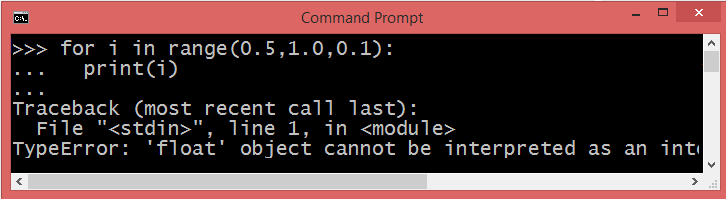If you provide a non-integer stop value, then it raises a TypeError: 'float' object cannot be interpreted as an integer .

However, you can implement an alternate way to get the range of floating numbers .

def floatRane(start, stop, step): i = start while i < stop: yield i i += step for i in floatRane(0.4, 0.7, 0.1): print(i)
output
0.4, 0.5, 0.6

## Python while loop using range() function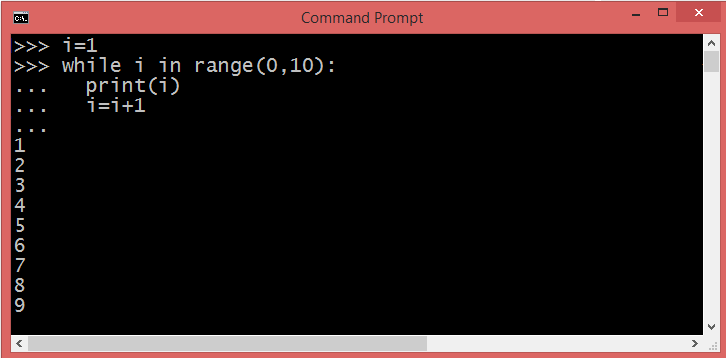## Concatenation of two range() functions using chain() method

The chain() method takes any number of iterables as arguments and "chains" them together.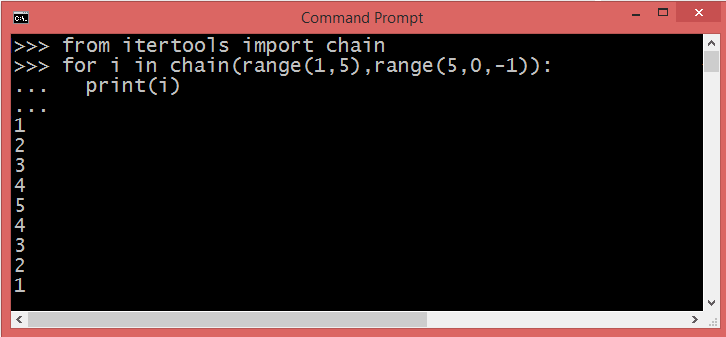## Accessing Python range() values using index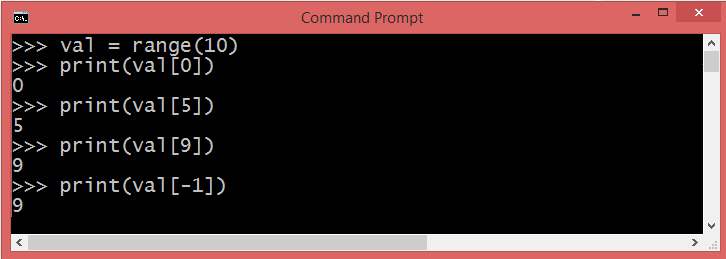## Python range() to List

The Python range type represents an immutable sequence of numbers, so it is possible to convert the output of a range() to the Python list.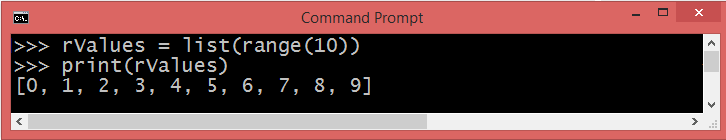### Conclusion

The Python range() function facilitates the creation of sequences of numbers, offering control over the start, end, and step size of the sequence. It efficiently generates values on demand, serving as a memory-efficient tool for looping and iteration tasks.